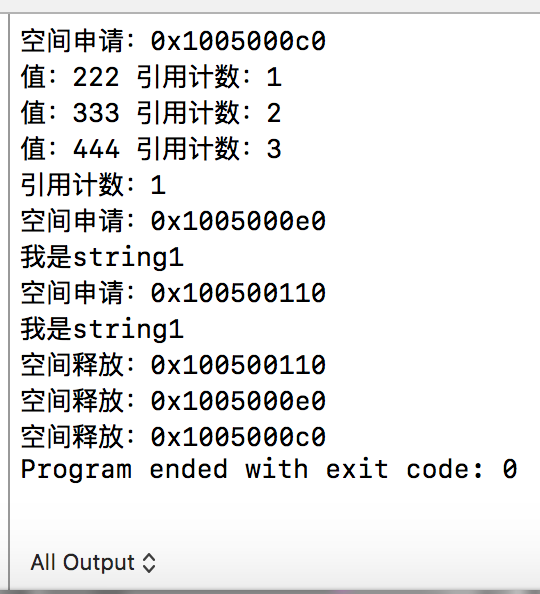# shared_ptr智能指针模板类的简单实现(c++11)

## 智能指针需要哪些组成部分

### 模拟指针行为

 T& operator*();//解引用重载
T* operator->();


#### 箭头运算符重载的特殊性

c++primer第五版上关于箭头运算符的原话是

## 代码

//
//  Shared_ptr.hpp
//  demo
//
//  Created by shiyi on 2016/12/10.
//

#ifndef Shared_ptr_Hpp
#define Shared_ptr_Hpp

#include <stdio.h>
#include <iostream>

using namespace std;

template <typename T>
class Shared_ptr
{
private:
size_t* m_count;
T* m_ptr;

public:
//构造函数
Shared_ptr() : m_ptr(nullptr), m_count(new size_t)
{}

Shared_ptr( T* ptr ) : m_ptr(ptr), m_count(new size_t)
{
cout<<"空间申请："<<ptr<<endl;
*m_count = 1;
}

//析构函数
~Shared_ptr()
{
--(*m_count);
if(*m_count == 0)
{
cout<<"空间释放："<<m_ptr<<endl;
delete m_ptr;
delete m_count;
m_ptr = nullptr;
m_count = nullptr;
}
}

//拷贝构造函数
Shared_ptr( const Shared_ptr& ptr )
{
m_count = ptr.m_count;
m_ptr = ptr.m_ptr;
++(*m_count);
}

//拷贝赋值运算符
void operator=( const Shared_ptr& ptr )
{
Shared_ptr(std::move(ptr));
}

//移动构造函数
Shared_ptr( Shared_ptr&& ptr ) : m_ptr(ptr.m_ptr), m_count(ptr.m_count)
{
++(*m_count);
}

//移动赋值运算符
void operator=( Shared_ptr&& ptr )
{
Shared_ptr(std::move(ptr));
}

//解引用运算符
T& operator*()
{
return *m_ptr;
}

//箭头运算符
T* operator->()
{
return m_ptr;
}

//重载布尔值操作
operator bool()
{
return m_ptr == nullptr;
}

T* get()
{
return m_ptr;
}

size_t use_count()
{
return *m_count;
}

bool unique()
{
return *m_count == 1;
}

void swap( Shared_ptr& ptr )
{
std::swap(*this, ptr);
}

};

#endif /* Shared_ptr_Hpp */

### 测试代码

#include "Shared_ptr.hpp"

using namespace std;

int main()
{
Shared_ptr<int> p1(new int);
*p1 = 222;
cout<<"值："<<*p1<<" 引用计数："<<p1.use_count()<<endl;
{
Shared_ptr<int> p2(p1);
*p2 = 333;
cout<<"值："<<*p2<<" 引用计数："<<p1.use_count()<<endl;

Shared_ptr<int> p3(p2);
*p3 = 444;
cout<<"值："<<*p3<<" 引用计数："<<p1.use_count()<<endl;
}

cout<<"引用计数："<<p1.use_count()<<endl;

Shared_ptr<string> q1(new string("我是string1"));
cout<<(*(q1)).c_str()<<endl;

Shared_ptr<string> q2(new string("我是string2"));
q2.swap(q1);
cout<<(*(q1)).c_str()<<endl;

return 0;
}


### 测试结果